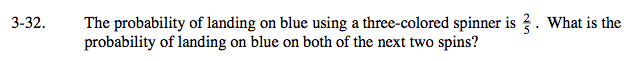### Home > AC > Chapter 14 > Lesson 14.3.1.2 > Problem3-32

3-32.What is the probability of flipping a coin twice and getting two heads in a row? How is this situation different?

$\text{The probability is }\frac{2}{5}\cdot\frac{2}{5}=0.16.$